Anzeige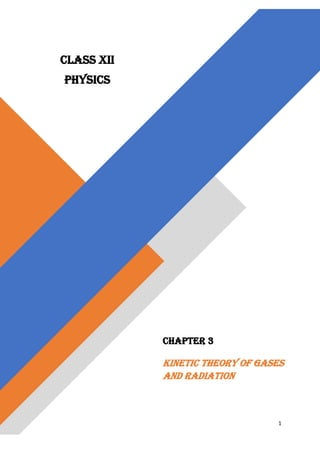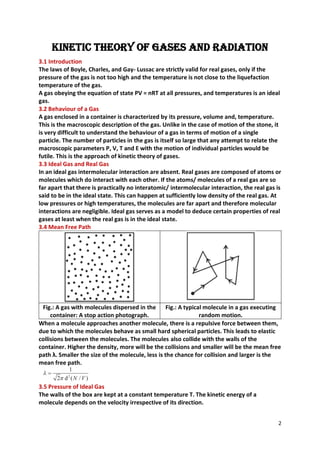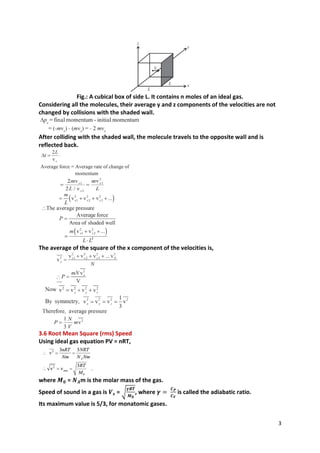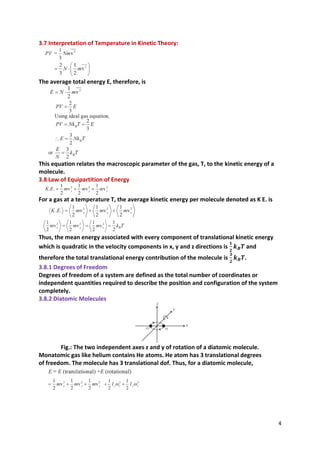Anzeige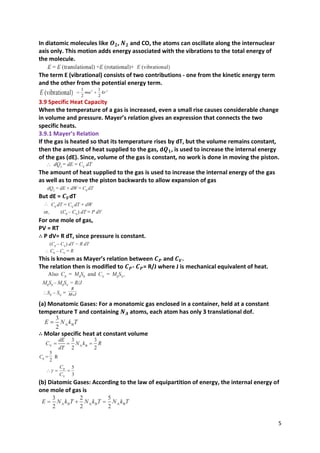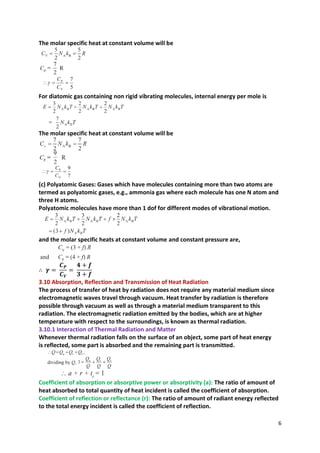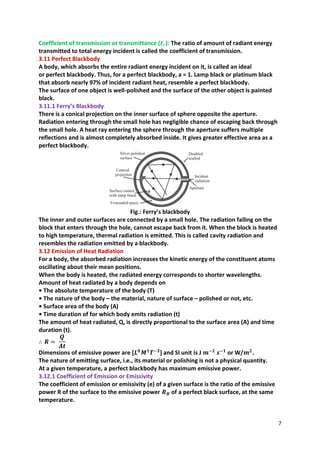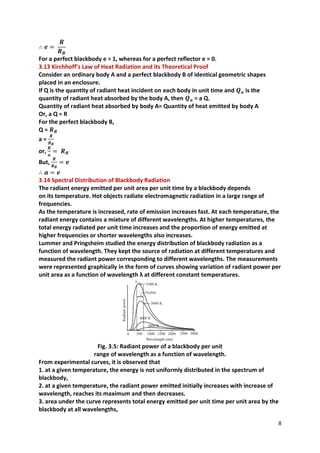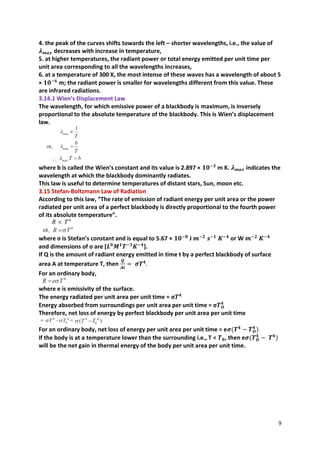Nächste SlideShare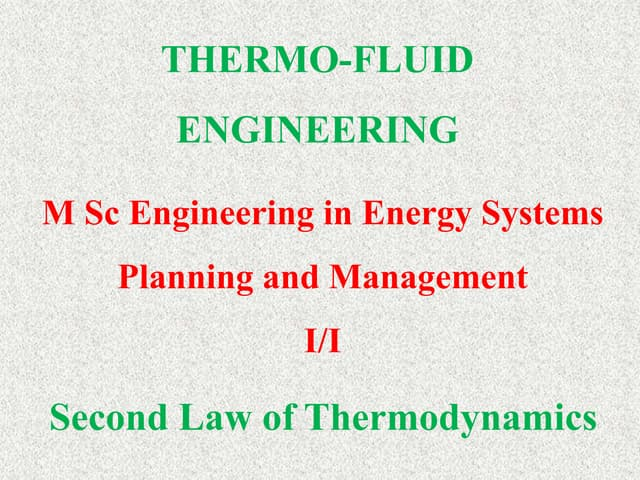second law
1 von 9
Anzeige

### PHYSICS CLASS XII Chapter 3 - Kinetic theory of gases and radiation

1. 1 CLASS XII PHYSICS CHAPTER 3 Kinetic Theory of Gases and Radiation
2. 2 Kinetic Theory of Gases and Radiation 3.1 Introduction The laws of Boyle, Charles, and Gay- Lussac are strictly valid for real gases, only if the pressure of the gas is not too high and the temperature is not close to the liquefaction temperature of the gas. A gas obeying the equation of state PV = nRT at all pressures, and temperatures is an ideal gas. 3.2 Behaviour of a Gas A gas enclosed in a container is characterized by its pressure, volume and, temperature. This is the macroscopic description of the gas. Unlike in the case of motion of the stone, it is very difficult to understand the behaviour of a gas in terms of motion of a single particle. The number of particles in the gas is itself so large that any attempt to relate the macroscopic parameters P, V, T and E with the motion of individual particles would be futile. This is the approach of kinetic theory of gases. 3.3 Ideal Gas and Real Gas In an ideal gas intermolecular interaction are absent. Real gases are composed of atoms or molecules which do interact with each other. If the atoms/ molecules of a real gas are so far apart that there is practically no interatomic/ intermolecular interaction, the real gas is said to be in the ideal state. This can happen at sufficiently low density of the real gas. At low pressures or high temperatures, the molecules are far apart and therefore molecular interactions are negligible. Ideal gas serves as a model to deduce certain properties of real gases at least when the real gas is in the ideal state. 3.4 Mean Free Path Fig.: A gas with molecules dispersed in the container: A stop action photograph. Fig.: A typical molecule in a gas executing random motion. When a molecule approaches another molecule, there is a repulsive force between them, due to which the molecules behave as small hard spherical particles. This leads to elastic collisions between the molecules. The molecules also collide with the walls of the container. Higher the density, more will be the collisions and smaller will be the mean free path λ. Smaller the size of the molecule, less is the chance for collision and larger is the mean free path. 3.5 Pressure of Ideal Gas The walls of the box are kept at a constant temperature T. The kinetic energy of a molecule depends on the velocity irrespective of its direction.
3. 3 Fig.: A cubical box of side L. It contains n moles of an ideal gas. Considering all the molecules, their average y and z components of the velocities are not changed by collisions with the shaded wall. After colliding with the shaded wall, the molecule travels to the opposite wall and is reflected back. The average of the square of the x component of the velocities is, 3.6 Root Mean Square (rms) Speed Using ideal gas equation PV = nRT, where 𝑴𝟎 = 𝑵𝑨m is the molar mass of the gas. Speed of sound in a gas is 𝑽𝒔 = √ 𝜸𝑹𝑻 𝑴𝟎 , where 𝜸 = 𝑪𝑷 𝑪𝑽 is called the adiabatic ratio. Its maximum value is 5/3, for monatomic gases.
4. 4 3.7 Interpretation of Temperature in Kinetic Theory: The average total energy E, therefore, is This equation relates the macroscopic parameter of the gas, T, to the kinetic energy of a molecule. 3.8 Law of Equipartition of Energy For a gas at a temperature T, the average kinetic energy per molecule denoted as K E. is Thus, the mean energy associated with every component of translational kinetic energy which is quadratic in the velocity components in x, y and z directions is 𝟏 𝟐 𝒌𝑩𝑻 and therefore the total translational energy contribution of the molecule is 𝟑 𝟐 𝒌𝑩𝑻. 3.8.1 Degrees of Freedom Degrees of freedom of a system are defined as the total number of coordinates or independent quantities required to describe the position and configuration of the system completely. 3.8.2 Diatomic Molecules Fig.: The two independent axes z and y of rotation of a diatomic molecule. Monatomic gas like helium contains He atoms. He atom has 3 translational degrees of freedom. The molecule has 3 translational dof. Thus, for a diatomic molecule,
5. 5 In diatomic molecules like 𝑶𝟐, 𝑵𝟐 and CO, the atoms can oscillate along the internuclear axis only. This motion adds energy associated with the vibrations to the total energy of the molecule. The term E (vibrational) consists of two contributions - one from the kinetic energy term and the other from the potential energy term. 3.9 Specific Heat Capacity When the temperature of a gas is increased, even a small rise causes considerable change in volume and pressure. Mayer’s relation gives an expression that connects the two specific heats. 3.9.1 Mayer’s Relation If the gas is heated so that its temperature rises by dT, but the volume remains constant, then the amount of heat supplied to the gas, d𝑸𝟏, is used to increase the internal energy of the gas (dE). Since, volume of the gas is constant, no work is done in moving the piston. The amount of heat supplied to the gas is used to increase the internal energy of the gas as well as to move the piston backwards to allow expansion of gas But dE = 𝑪𝑽dT For one mole of gas, PV = RT ∴ P dV= R dT, since pressure is constant. This is known as Mayer’s relation between 𝑪𝑷 and 𝑪𝑽. The relation then is modified to 𝑪𝑷- 𝑪𝑷= R/J where J is mechanical equivalent of heat. (a) Monatomic Gases: For a monatomic gas enclosed in a container, held at a constant temperature T and containing 𝑵𝑨 atoms, each atom has only 3 translational dof. ∴ Molar specific heat at constant volume (b) Diatomic Gases: According to the law of equipartition of energy, the internal energy of one mole of gas is
6. 6 The molar specific heat at constant volume will be For diatomic gas containing non rigid vibrating molecules, internal energy per mole is The molar specific heat at constant volume will be (c) Polyatomic Gases: Gases which have molecules containing more than two atoms are termed as polyatomic gases, e.g., ammonia gas where each molecule has one N atom and three H atoms. Polyatomic molecules have more than 1 dof for different modes of vibrational motion. and the molar specific heats at constant volume and constant pressure are, ∴ 𝜸 = 𝑪𝑷 𝑪𝑽 = 𝟒 + 𝒇 𝟑 + 𝒇 3.10 Absorption, Reflection and Transmission of Heat Radiation The process of transfer of heat by radiation does not require any material medium since electromagnetic waves travel through vacuum. Heat transfer by radiation is therefore possible through vacuum as well as through a material medium transparent to this radiation. The electromagnetic radiation emitted by the bodies, which are at higher temperature with respect to the surroundings, is known as thermal radiation. 3.10.1 Interaction of Thermal Radiation and Matter Whenever thermal radiation falls on the surface of an object, some part of heat energy is reflected, some part is absorbed and the remaining part is transmitted. Coefficient of absorption or absorptive power or absorptivity (a): The ratio of amount of heat absorbed to total quantity of heat incident is called the coefficient of absorption. Coefficient of reflection or reflectance (r): The ratio of amount of radiant energy reflected to the total energy incident is called the coefficient of reflection.